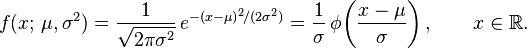# Probability over an interval in a Normal Distribution?

## Homework Statement

In a photographic process, the developing time of prints may be looked upon as
a random variable which is normally distributed with a mean of 16.28 seconds
and a standard deviation of 0.12 second. Find the probability that it will take
anywhere from 16.00 to 16.50 seconds to develop one of the prints.

## Homework Equationssigma = std dev = 0.12
mu = mean = 16.28

## The Attempt at a Solution

I *think* I know what I need to do, just dont know how to do it:

So I have the Guassian Distribution formula (with std dev & mean plugged in) as my Probability Density Function.
I need to find the area under the Cumulative Distribution Function over the interval 16 to 16.50.
Because the integrals in CDFs are evaluated from -inf to a, I need to subtract the (integral of CDF from -inf to 16) from the (integral of CDF from -inf to 16.50), and that will be my answer.

But I don't know how to (A) Get the CDF, (B) Evaluate the CDF integral.

Ive tried reading up on the net but I'm not following the theory, can someone please show me how to do this? Thank you!

## Answers and Replies

Staff Emeritus
Homework Helper
Yes, that is entirely correct. However, you cannot solve the CDF integral explicitly or find the CDF, since the integral involved is unsolvable.

What you need to do is find the CDF is tables or using computer programs. Are you following a course in probability? Then you should have been given a table with the CDF of the standard normal distribution. Otherwise, see http://www.pavementinteractive.org/index.php?title=Normal_Distribution where a table has been given at the bottom of the page...

Of course, these tables and many of the tables you know are only of the standard normal distribution (that is: mean=0 and stdev=1). So you'll need to convert your probability to a probability involving the standard normal distribution...

Homework Helper

## Homework Statement

In a photographic process, the developing time of prints may be looked upon as
a random variable which is normally distributed with a mean of 16.28 seconds
and a standard deviation of 0.12 second. Find the probability that it will take
anywhere from 16.00 to 16.50 seconds to develop one of the prints.

## Homework Equationssigma = std dev = 0.12
mu = mean = 16.28

## The Attempt at a Solution

I *think* I know what I need to do, just dont know how to do it:

So I have the Guassian Distribution formula (with std dev & mean plugged in) as my Probability Density Function.
I need to find the area under the Cumulative Distribution Function over the interval 16 to 16.50.
Because the integrals in CDFs are evaluated from -inf to a, I need to subtract the (integral of CDF from -inf to 16) from the (integral of CDF from -inf to 16.50), and that will be my answer.

But I don't know how to (A) Get the CDF, (B) Evaluate the CDF integral.

Ive tried reading up on the net but I'm not following the theory, can someone please show me how to do this? Thank you!

Yes, that's correct. The CDF is the integral:

$$F(x, \mu, \sigma^2) = \int \limits_{-\infty} ^ x f(t, \mu, \sigma^2)dt = \Phi \left( \frac{x - \mu}{\sigma} \right) = \frac{1}{2} \left( 1 + \mbox{erf} \left( \frac{x - \mu}{\sqrt{2}\sigma} \right) \right)$$

There should be some table of value at the back of your book of $$\Phi$$, or erf function.

Or you can even get it online:
• http://www.geophysik.uni-muenchen.de/~malservisi/GlobaleGeophysik2/erf_tables.pdf" [Broken]
• http://www.math.unb.ca/~knight/utility/NormTble.htm" [Broken]

Cheers,

Last edited by a moderator:
Homework Helper
Dearly Missed
You need access to tables or computer-generated values of the normal cumulative. Besides the sources listed in the other responses, you can find the normal cumulative on some of the better hand-held calculators. It is also a built-in function in EXCEL and other, similar spreadsheets.

R.G. Vickson

Homework Helper
Gold Member
You need access to tables or computer-generated values of the normal cumulative. Besides the sources listed in the other responses, you can find the normal cumulative on some of the better hand-held calculators. It is also a built-in function in EXCEL and other, similar spreadsheets.

R.G. Vickson

Another refugee from sci.math, eh? Welcome to PF Ray (I assume that's you).

--Lynn